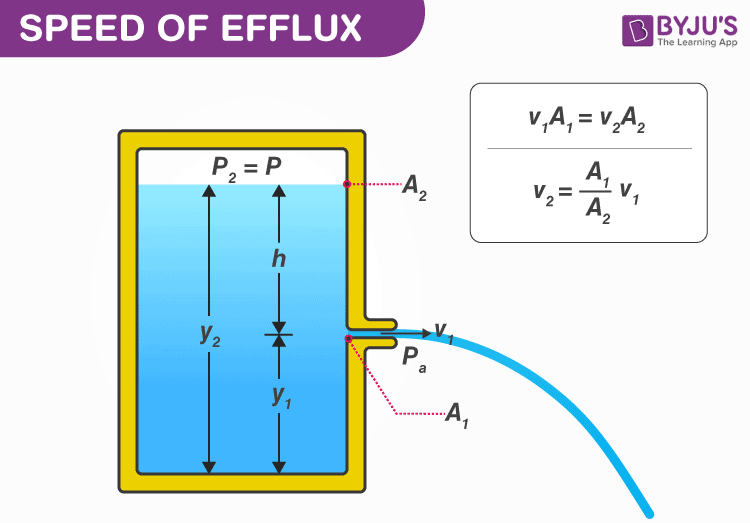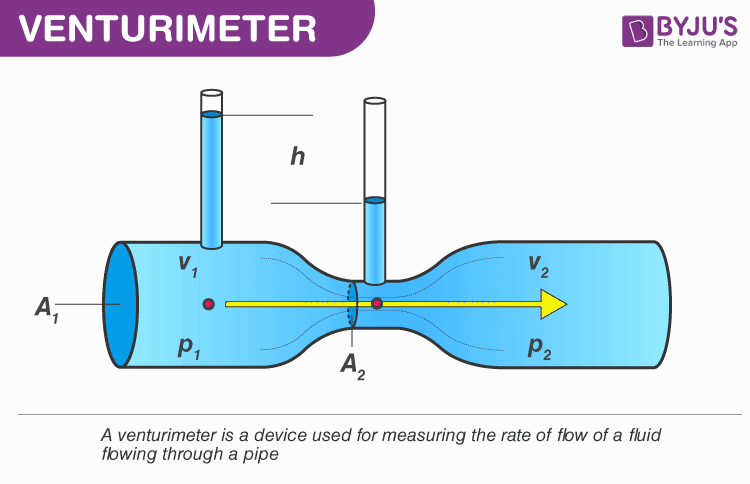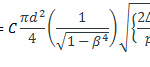# Speed Of Efflux - Venturimeter And Its Working Equation

In this article, we will discuss the speed of efflux, its calculation, Torricelli’s law, its derivation, venturimeter, its working principle and its working equation.

## Torricelli’s Law

As per the Torricelli’s law for Newtonian fluids, the density of efflux of a fluid passing through a sharp-edged hole at the bottom of a tank filled with the fluid to a depth of h is the same as the speed that a body would acquire in a freely falling condition when falling from a height h.

### DerivationConsider a tank with a small hole in its side at a height y1 from the bottom, containing a liquid of density ρ. The air above the liquid is at pressure P and its surface is at height y2.

From the equation of continuity, we can write

A1v1 = A2v2

Or v2 = A1v1/A2  ………………………….(1)

If the cross-sectional area of the tank A2 is much larger than that of the hole (A2 >>A1), then we may take the fluid to be approximately at rest at the top, i.e. v2 = 0. Now applying the Bernoulli equation at points 1 and 2 and noting that at the hole P1 = Pa (the atmospheric pressure), we have

Pa + 1/2ρv12+ ρgy1 = Pb + 1/2ρv22 + ρgy2 ……………………………(2)

Here, let the difference in the height = h (as shown in the figure) = y1-y2

From equation 1 and 2, we have

$$\begin{array}{l}v_{1}=\sqrt{\frac{2}{\rho}}[(p-p_{a})+ \rho gh]\end{array}$$

## Venturimeter

Venturimeter is a flow measurement instrument. Here, a converging section of a pipe is used to give an increase in the flow velocity and a corresponding pressure drop from which the flow rate of the fluid is deduced based on the Bernoulli’s equation.Let us consider the figure shown above. Here we can see the block diagram of a Venturimeter. Here we can see a small converging part, a throat and a diverging part.  Here, we apply the Bernoulli’s equation between the inline section and the throat section. The pressure difference is measured using a manometer.

P1 + 1/2ρV12 = P2 + 1/2ρV22

Where P1 is the pressure in the inline section and p2 is the pressure in the throat section, V1 is the velocity of the fluid passing through the inline section and v2 and the velocity of the fluid passing through the throat section and ρ is the density of the liquid.

Now, from the equation of continuity, we can say

Volumetric flow rate = V = ¼ πD2u1 = ¼ πd2u2

Where V is the volumetric flow rate of the liquid, D is the diameter of the pipe and d is the diameter of the throat.

Combining the two equations, we can writeWhere, β is the ratio of diameters, d/D.

Here, we introduce a venture coefficient (C) considering the loss due to pipe friction and change in the total pressure,Where Δp is the pressure difference and C is the coefficient of Venturimeter.

Stay tuned with BYJU’S for more such interesting articles. Also, register to “BYJU’S – The Learning App” for loads of interactive, engaging Physics-related videos and an unlimited academic assist.

## Understanding Continuity EquationTest Your Knowledge On Efflux Venturimeter!